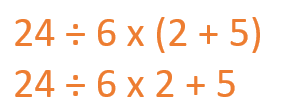Smartick is a fun way to learn math!Nov09

# How to Solve Combined Operations

In this post, we will learn the order that needs to be followed to solve combined operations: addition, subtraction, multiplication, and division. The combined operations cannot be performed at random, an order must be followed.• Step 1: Perform operations that are within parenthesis.

For example: 3 x (2 + 4).

First, we do the operation inside the parenthesis: 2 + 4 = 6.

Then we perform the operation: 3 x 6 = 18.

• Step 2: perform multiplication and division, always from left to right.

For example: 24 ÷ 6 x 2.

First, we perform the division because it is further to the left than the multiplication:  24 ÷ 6 = 4.

Then we do the multiplication: 4 x 2 = 8.

• Step 3: Finally, do additions and subtractions.

For example: 2 + 3 x 5.

First, we do the multiplication: 3 x 5 = 15.

Then we take the sum: 2 + 15 = 17.

## 6 + (8-3) x 2

First we complete the operation in parenthesis: 8 – 3 = 5.

From here, we have: 6 + 5 x 2.

Now we do the multiplication: 5 x 2 = 10.
And finally we have addition: 6 + 10 = 16.

## 21 ÷ 3 + 7 x 4

The first operation to be completed is in the parenthesis but in this case, there are none.
The next thing to do is multiplication and division: 21/3 = 7 and on the other 7 x 4 = 28
Now we only have addition to complete: 7 + 28 = 35

Click here if you want to learn Tricks to Solve Combined Operations! Or enter Smartick and continue to learn mathematics.

Fun is our brain’s favorite way of learning
Diane Ackerman
Smartick is a fun way to learn math
• 15 fun minutes a day
• Millions of students since 2009• JamesJun 02 2022, 2:00 PM

It’s maths l love ❤ mathematics is a good subject

• Brithany AnglinJan 19 2021, 3:13 PM

This has been an amazing experience guys, thanks so much for this page it has helped me tremendously and I truly appreciate it.

• Paulin6Feb 08 2023, 1:42 PM

It’s really amazing to know how to tackel maths questions easily

• Crizza Leah CalanaoJun 05 2019, 10:16 AM

Thanks for more 😘

• ALNov 23 2017, 7:51 PM

Very good explanation！Thanks!